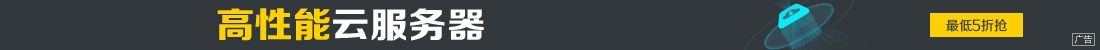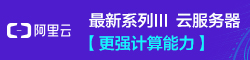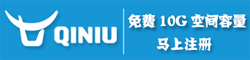# Python制作微信好友背景墙教程（附完整代码）

••## 引言Python版本：3.7.3

## 代码实现

### 准备工作

```from wxpy import *
import PIL.Image as Image
import os
import sys
```

### 获取并存储好友头像信息

```# 初始化机器人，扫码登陆微信，适用于Windows系统
bot = Bot()

# # Linux系统，执行登陆请调用下面的这句
# bot = Bot(console_qr=2, cache_path="botoo.pkl"```

```# 获取当前路径信息
curr_dir = get_dir(sys.argv)
# 如果FriendImgs目录不存在就创建一个
if not os.path.exists(curr_dir + "FriendImgs/"):
os.mkdir(curr_dir + "FriendImgs/")```

```my_friends = bot.friends(update=True)
# 获取好友头像信息并存储在FriendImgs目录中
n = 0
for friend in my_friends:
friend.get_avatar(curr_dir + "FriendImgs/" + str(n) + ".jpg")
n = n + 1```### 生成微信好友墙

`image = Image.new("RGB", (650, 650))`

`img = Image.open(curr_dir + "FriendImgs/" + file_names)`

`img = img.resize((50, 50), Image.ANTIALIAS)`

`image.paste(img, (x * 50, y * 50))`

`img = image.save(curr_dir + "WeChat_Friends.jpg")`

```# 准备生成微信好友头像墙的尺寸
image = Image.new("RGB", (650, 650))

# 定义初始图片的位置
x = 0
y = 0

# 获取下载的头像文件
curr_dir = get_dir(sys.argv)
ls = os.listdir(curr_dir + 'FriendImgs')

# 遍历文件夹的图片
for file_names in ls:
try:
# 依次打开图片
img = Image.open(curr_dir + "FriendImgs/" + file_names)
except IOError:
continue
else:
# 重新设置图片的大小
img = img.resize((50, 50), Image.ANTIALIAS)
# 将图片粘贴到最终的照片墙上
image.paste(img, (x * 50, y * 50))
# 设置每一行排13个图像
x += 1
if x == 13:
x = 0
y += 1
# 保存图片为WeChat_Friends.jpg
img = image.save(curr_dir + "WeChat_Friends.jpg```(这里展示的图片做了模糊处理)

## 后记